# Verified Analysis of Random Binary Tree Structures

## Abstract

This work is a case study of the formal verification and complexity analysis of some famous probabilistic algorithms and data structures in the proof assistant Isabelle/HOL. In particular, we consider the expected number of comparisons in randomised quicksort, the relationship between randomised quicksort and average-case deterministic quicksort, the expected shape of an unbalanced random Binary Search Tree, the randomised binary search trees described by Martínez and Roura, and the expected shape of a randomised treap. The last three have, to our knowledge, not been analysed using a theorem prover before and the last one is of particular interest because it involves continuous distributions.

## Introduction

This paper conducts verified analyses of a number of classic probabilistic algorithms and data structures related to binary search trees. It is part of a continuing research programme to formalise classic data structure analyses [28,29,30]. The key novel contributions of the paper are readable (with one caveat, discussed in the conclusion) formalised analyses of

• the precise expected number of comparisons in randomised quicksort,

• the relationship between the average-case behaviour of deterministic quicksort and the result distribution of randomised quicksort,

• the expected path length and height of a random binary search tree,

• the expected shape of a treap, which involves continuous distributions,

• the randomised binary search trees due to Martínez and Roura (‘MR trees’) .

The above algorithms are shallowly embedded and expressed using the Giry monad, which allows for a natural and high-level presentation. All verifications were carried out in Isabelle/HOL [31, 32].

After an introduction to the representation of probability theory in Isabelle/HOL, the core content of the paper consists of four sections that analyse quicksort, random binary search trees, randomised treaps, and MR trees, respectively. The corresponding formalisations can be found in the Archive of Formal Proofs [8,9,10, 16]. We then conclude with an overview of related work and a summary of what we achieved and what could be improved.

This paper is a revised and extended version of one presented at ITP 2019 . The most substantial difference is that the previous version did not contain a discussion of MR trees.

## Probability Theory in Isabelle/HOL

### Measures and Probability Mass Functions

The foundation for measure theory (and thereby probability theory) in Isabelle/HOL was laid by Hölzl . This approach is highly general and flexible, allowing measures with uncountably infinite support (e. g. normal distributions on the reals) and has been used for a number of large formalisation projects related to randomisation, e. g. Ergodic theory , compiling functional programs to densities , Markov chains and decision processes , cryptographic algorithms , and probabilistic primality tests .

Initially we shall only consider probability distributions over countable sets. In Isabelle, these are captured as probability mass functions (PMFs). A PMF is simply a function that assigns a probability to each element, with the property that the probabilities are non-negative and sum up to 1. For any HOL type $$\alpha$$, the type $$\alpha \ \textit{pmf}$$ denotes the type of all probability distributions over values of type $$\alpha$$ with countable support.

Working with PMFs is quite pleasant, since we do not have to worry about measurability of sets or functions. Since everything is countable, we can always choose the power set as the measurable space, which means that any function and any set is always trivially measurable.

Later, however, we will also need to be able to express continuous distributions. For these, there exists a type $$\alpha \ \textit{measure}$$, which describes a measure-theoretic measure over elements of type $$\alpha$$. Such a measure is formally defined as a triple consisting of a carrier set $$\varOmega$$, a $$\sigma$$-algebra on $$\varOmega$$ (which we call the set of measurable sets), and a measure function $$\mu : \alpha \rightarrow \textit{ennreal}$$, where $$\textit{ennreal}$$ is the type of extended non-negative real numbers $$\mathbb R_{{\ge }0} \cup \{\infty \}$$. Of course, since we only consider probability measures here, our measures will always return values between 0 and 1. To our knowledge, as of 2019, Isabelle/HOL and Lean are the only systems that have a sufficiently general library of measure theory to handle such continuous distributions.

One problem with these general measures (which are only relevant for Sect. 5) is that we often need to annotate the corresponding $$\sigma$$-algebras and prove that everything we do with a distribution is in fact measurable. These details are unavoidable on a formal level, but typically very uninteresting to a human: There is usually a ‘natural’ choice for these $$\sigma$$-algebras and any set or operation that can be written down explicitly is typically measurable in some adequate sense. For the sake of readability, we will therefore omit everything related to measurability in this presentation.

Table 1 gives an overview of the notation that we use for PMFs and general measures. We allow ourselves some notational freedom in this paper: For instance, some of the operations on general measures (such as $${ uniform\_measure}$$) actually require another additional parameter to specify the underlying measurable space, which we omitted here since that parameter is clear from the context and would only make the presentation less readable.

Specifying probabilistic algorithms compositionally requires a way to express sequential composition of random choice. The standard way to do this is the Giry monad . A detailed explanation of this (especially in the context of Isabelle/HOL) can be found in an earlier paper by Eberl et al. . For the purpose of this paper, we only need to know that the Giry monad provides functions

\begin{aligned} \text {return} \,{:}{:}\, \alpha \rightarrow \alpha \ \text {pmf} \quad \text {bind} \,{:}{:}\, \alpha \ \text {pmf} \rightarrow (\alpha \rightarrow \beta \ \text {pmf} )\rightarrow \beta \ \text {pmf} \end{aligned}

(and analogously for $$\alpha \ \textit{measure}$$) where $$\textit{return}\ x$$ gives us the singleton distribution where x is chosen with probability 1 and $$\textit{bind}\ p\ f$$ composes two distributions in the intuitive sense of randomly choosing a value x according to the distribution p and then returning a value randomly chosen according to the distribution f(x).

For better readability, Isabelle supports a Haskell-like do-notation as syntactic sugar for $$\textit{bind}$$ operations where e. g.

\begin{aligned} \text {bind} \ A\ (\uplambda x.\ \text {bind} \ B\ (\uplambda y.\ \text {return} \ (x + y))) \end{aligned}

can be written succinctly as

\begin{aligned} \mathbf{do} \ \{x \leftarrow A;\ y \leftarrow B;\ \text {return} \ (x + y)\}\ . \end{aligned}

## Quicksort

We now show how to define and analyse quicksort [17, 37] (in its functional representation) within this framework. Since all of the randomisation is discrete in this case, we can restrict ourselves to PMFs for the moment.

For the sake of simplicity (and because it relates to binary search trees, which we will treat later), we shall only treat the case of sorting lists without repeated elements. (See the end of this section for further discussion of this point.)

As is well known, quicksort has quadratic worst-case performance if the pivot is chosen poorly. Using the true median as the pivot would solve this, but is impractical. Instead, a simple alternative is to choose the pivot randomly, which is the variant that we shall analyse first.

### Randomised Quicksort

Intuitively, the good performance of randomised quicksort can be explained by the fact that a random pivot will usually not be among the most extreme values of the list, but somewhere in the middle, which means that, on average, the size of the lists is reduced significantly in every recursion step.

To make this more rigorous, let us look at the definition of the algorithm in Isabelle. Note that the algorithm returns not only the result, but a pair of the result and the number of comparisons that it made in total:

### Definition 1

(Randomised quicksort)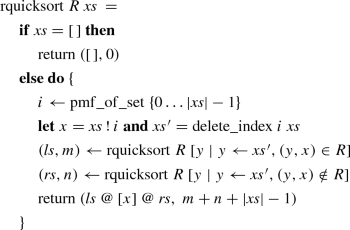Here, $${\mathbin {@}}$$ denotes list concatenation and $$\textit{xs}\mathbin {!}i$$ denotes the ith element of the list $$\textit{xs}$$, where $$0 \le i < |\textit{xs}|$$. The delete_index function removes the ith element of a list, and the parameter R is a linear ordering represented as a set of pairs.

It is easy to prove that all of the lists that can be returned by the algorithm are sorted w. r. t. R. The base case makes 0 comparisons and the recursive case makes $$|\textit{xs}| - 1 + m + n$$ comparisons, where m and n are the numbers of comparisons made by the recursive calls. This could easily be encapsulated in a resource monad (as we have done elsewhere  for more complex code), but it is not worth the effort in this case.

For an element x and some list $$\textit{xs}$$, we call the number of elements of $$\textit{xs}$$ that are smaller than x the rank of x w. r. t. $$\textit{xs}$$. In lists with distinct elements, each element can clearly be uniquely identified by either its index in the list or its rank w. r. t. that list, so choosing an element uniformly at random, choosing an index uniformly at random, or choosing a rank uniformly at random are all interchangeable.

In the above algorithm, the length of $$\textit{ls}$$ is simply the rank r of the pivot, and the length of $$\textit{rs}$$ is simply $$|\textit{xs}| - 1 - r$$, so choosing the pivot uniformly at random means that the length of $$\textit{ls}$$ is also distributed uniformly between 0 and $$|\textit{xs}| - 1$$. From this, we can see that the distribution of the number of comparisons does not actually depend on the content of the list or the ordering R at all, but only on the length of the list, and we can find the following recurrence for it:

### Definition 2

(Cost of randomised quicksort)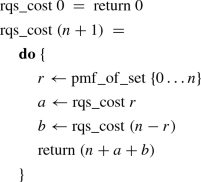We then have the following relationship between $$\text {rquicksort}$$ and $$\text {rqs}\_\text {cost}$$:

### Theorem 1

Let R be a linear ordering on some set A and let $$\textit{xs}$$ be a list of distinct elements from A. Then:

\begin{aligned} \mathrm{map}\_\mathrm{pmf}\ \text {snd} \ (\text {rquicksort} \ R\ \textit{xs})\ =\ \mathrm{rqs}\_\mathrm{cost}\ |\textit{xs}| \end{aligned}
(1)

In other words: Projecting out the number of comparisons from our cost-aware randomised quicksort yields the distribution given by $$\textit{rqs}\_\textit{cost}$$.

### Proof

The proof is a fairly simple one, but it is instructive to show it in some detail nevertheless in order to convey to the reader how such proofs can be done in a formal setting. We will rewrite the distributions in question step by step, with each intermediate expression closely mirroring the formal proof in Isabelle.

We show (1) by induction over $$\textit{xs}$$, following the recursive definitions of $$\textit{rquicksort}$$ and $$\textit{rqs}\_\textit{cost}$$. The base case—an empty list—is obvious. For the induction step, we fix a non-empty list $$\textit{xs}$$ and assume as the induction hypothesis that (1) already holds for any proper sublist of $$\textit{xs}$$. From this, we now need to show that (1) holds for $$\textit{xs}$$ as well.

To do this, we first consider the left-hand side of (1), unfold one step of the recursive definition of $$\textit{rquicksort}$$, and use the fact that $$\textit{map}\_\textit{pmf}$$  distributes over $$\textit{bind}$$ and $$\textit{return}$$ to arrive at the following expression: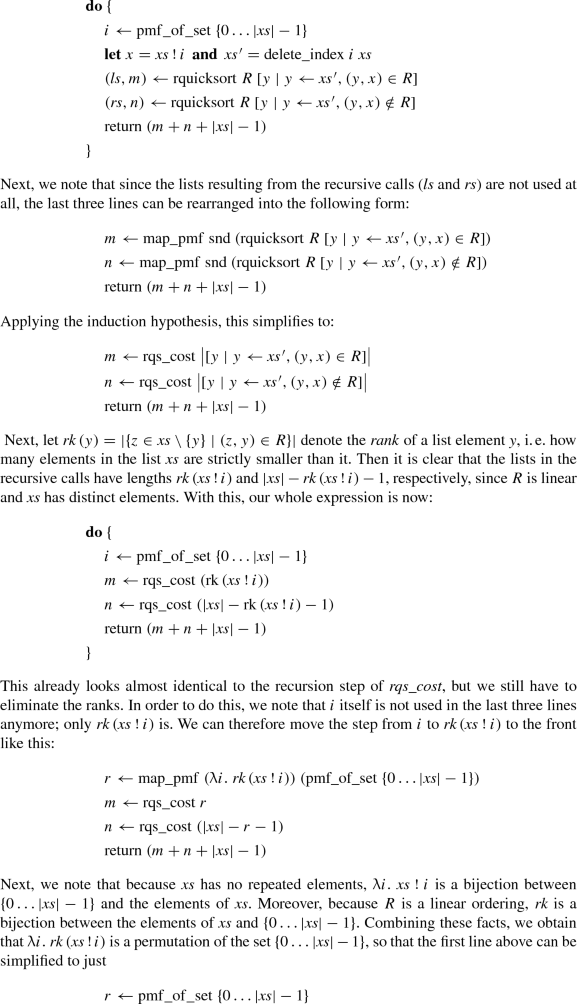since the uniform distribution is invariant under permutation. Comparing this to the recursive step in the definition of $$\textit{rqs}\_\textit{cost}$$, we note that this is precisely what we had to show. $$\square$$

Next, we will attempt to determine the expected value of $$\textit{rqs}\_\textit{cost}$$, which we shall denote by Q(n). The recursive definition of $$\textit{rqs}\_\textit{cost}$$ directly leads us to the recurrence

\begin{aligned} Q(n+1) = n + \frac{1}{n+1}\left( \sum _{i=0}^n Q(i) + Q(n - i)\right) \ , \end{aligned}

or, equivalently,

\begin{aligned} Q(n+1) = n + \frac{2}{n+1}\left( \sum _{i=0}^n Q(i)\right) \ . \end{aligned}

This is often called the quicksort recurrence. Cichoń  gave a simple way of solving this by turning it into a linear recurrence

\begin{aligned} \frac{Q(n+1)}{n+2} = \frac{2n}{(n+1)(n+2)} + \frac{Q(n)}{n+1}\ , \end{aligned}

which gives us

\begin{aligned} \frac{Q(n)}{n+1} = 2\sum _{k=1}^n \frac{k-1}{k(k+1)} = 4 H_{n+1} - 2 H_n - 4 \end{aligned}

by telescoping and thereby the closed-form solution

\begin{aligned} Q(n) = 2 (n+1) H_n - 4n\ , \end{aligned}

where $$H_n$$ is the nth harmonic number. We can use the well-known asymptotics $$H_n \sim \ln n + \gamma$$ (where $$\gamma \approx 0.5772$$ is the Euler–Mascheroni constant) from the Isabelle library and obtain that the expected number of comparisons fulfils $$Q(n) \sim 2 n \ln n$$.

Remember, however, that we only considered lists with no repeated elements. If there are repeated elements, the performance of the above algorithm can deteriorate to quadratic time. This can be fixed easily by using a three-way partitioning function instead, although this makes things slightly more complicated since the number of comparisons made now depends on the content of the list and not just its length. The only real difference in the cost analysis is that the lists in the recursive call no longer simply have lengths r and $$n - r - 1$$, but can also be shorter if the pivot is contained in the list more than once. We can still show that the expected number of comparisons is at most Q(n) in much the same way as before (and our entry  in the Archive of Formal Proofs does contain that proof), but we shall not go into more detail here.

Comparing our proof to those in the literature, note that both Cormen et al.  and Knuth  also restrict their analysis to distinct elements. Cormen et al. use a non-compositional approach with indicator variables and only derive the logarithmic upper bound, whereas Knuth’s analysis counts the detailed number of different operations made by a particular implementation of the algorithm in MIX. His general approach is very similar to the one presented here.

### Average-Case of Non-Randomised Quicksort

The above results carry over directly to the average-case analysis of non-randomised quicksort (again, we will only consider lists with distinct elements). Here, the pivot is chosen deterministically; we always choose the first element for simplicity. This gives us the following definitions of quicksort and its cost:

### Definition 3

(Deterministic quicksort and its cost)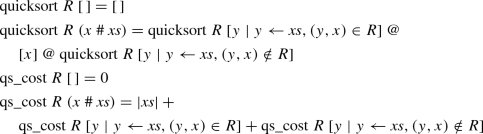Interestingly, the number of comparisons made on a randomly-permuted input list has exactly the same distribution as the number of comparisons in randomised quicksort from before. The underlying idea is that when randomly permuting the input, the randomness can be ‘deferred’ to the first point where an element is actually inspected, which means that choosing the first element of a randomly-permuted list as the pivot is equivalent to choosing the pivot uniformly at random.

The formal proof of this starts by noting that choosing a random permutation of a non-empty finite set A is the same as first choosing the first list element $$x\in A$$ uniformly at random and then choosing a random permutation of $$A\setminus \{x\}$$ as the remainder of the list, allowing us to pull out the pivot selection. Then, we note that taking a random permutation of $$A\setminus \{x\}$$ and partitioning it into elements that are smaller and bigger than x is the same as first partitioning the set $$A\setminus \{x\}$$ into $$\{y\in A\setminus \{x\} \mid (y,x)\in R\}$$ and $$\{y\in A\setminus \{x\}\mid (y,x)\notin R\}$$ and choosing independent random permutations of these sets.

This last step, which interchanges partitioning and drawing a random permutation, is probably the most crucial one and one that we will need again later, so we present the corresponding lemma in full here. Let $$\textit{partition}\ P\ \textit{xs}$$ be the function that splits the list $$\textit{xs}$$ into the pair of sub-sequences that satisfy (resp. do not satisfy) the predicate P. Then, we have:

### Lemma 1

(Partitioning a randomly permuted list) Let A be a finite set. Then: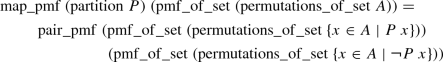Here, the function $$\textit{permutations}\_\textit{of}\_\textit{set}\ A$$ returns the set of all permutations of the given finite set A, i. e. the set of all lists that contain each element of A exactly once. The lemma is easily proven directly by extensionality, i. e. fixing permutations $$\textit{xs}$$ of $$\{x\in A \mid P\ x\}$$ and $$\textit{ys}$$ of $$\{x\in A \mid \lnot P\ x\}$$, computing their probabilities in the two distributions and noting that they are the same.

With this, the proof of the following theorem is just a straightforward induction on the recursive definition of $$\textit{rqs}\_\textit{cost}$$:

### Theorem 2

(Cost distribution of randomised quicksort) For every linear order R on a finite set A, we have:

\begin{aligned} \mathrm{map}\_\mathrm{pmf}\ (\mathrm{qs}\_\mathrm{cost}\ R)\ (\mathrm{pmf}\_\mathrm{of}\_\mathrm{set}\ (\mathrm{permutations}\_\mathrm{of}\_\mathrm{set}\ A)) = \mathrm{rqs}\_\mathrm{cost}\ |A| \end{aligned}

Thus, the cost distribution of deterministic quicksort applied to a randomly permuted list (with distinct elements) is the same as that of randomised quicksort applied to any list of the same size (with distinct elements). In particular, the asymptotic results about the expectation of $$\textit{rqs}\_\textit{cost}$$ carry over directly.

## Random Binary Search Trees

### Preliminaries

We now turn to another average-case complexity problem that is somewhat related to quicksort, though not in an obvious way. We consider node-labelled binary trees, defined by the algebraic datatype

\begin{aligned} \mathbf{datatype} \ \alpha \ \text {tree} = \text {Leaf} \ |\ \text {Node} \ (\alpha \ \text {tree} )\ \alpha \ (\alpha \ \text {tree} )\ . \end{aligned}

We denote $$\textit{Leaf}$$ by $$\langle \rangle$$ and $$\textit{Node}\ l\ x\ r$$ by $$\langle l, x, r\rangle$$. Given some linear ordering on the values at the nodes, we say that the tree is a binary search tree (BST) if, for every node with some element x, all of the values in the left sub-tree are smaller than x and all of the values in the right sub-tree are larger than x.

Inserting elements can be done by performing a search and, if the element is not already in the tree, adding a node at the leaf at which the search ends. We denote this operation by $$\textit{bst}\_\textit{insert}$$. Note that these are simple, unbalanced BSTs and our analysis will focus on what happens when elements are inserted into them in random order. We call the tree that results from adding elements of a set A to an initially empty BST in random order a random BST. This can also be seen as a kind of ‘average-case’ analysis of BSTs.

To analyse random BSTs, let us first examine what happens when we insert a list of distinct elements into an empty BST from left to right; formally:

### Definition 4

(Inserting a list of elements into a BST)

\begin{aligned} \text {bst} \_\text {of} \_\text {list} \ \textit{xs}\ =\ \text {fold} \ \text {bst} \_\text {insert} \ \textit{xs}\ \langle \rangle \end{aligned}

Let x be the first element of the list. This element will become the root of the tree and will never move again. Similarly, the next element will become either the left or right child of x and will then also never move again and so on. It is also clear that no elements greater than x will end up in the left sub-tree of x at any point in the process, and no elements smaller than it in its right sub-tree. This leads us to the following recurrence for $$\textit{bst}\_\textit{of}\_\textit{list}$$:

### Lemma 2

(Recurrence for $$\textit{bst}\_\textit{of}\_\textit{list}$$)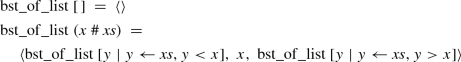We can now formally define our notion of ‘random BST’:

### Definition 5

(Random BSTs)

\begin{aligned}&\mathrm{rbst}\ A\ = \text {map} \_\text {pmf} \ \text {bst} \_\text {of} \_\text {list} \ (\text {pmf} \_\text {of} \_\text {set} \ (\text {permutations} \_\text {of} \_\text {set} \ A)) \end{aligned}

By re-using Lemma 1, we easily get the following recurrence:

### Lemma 3

(A recurrence for random BSTs)We can now analyse some of the properties of such a random BST. In particular, we will look at the expected height and the expected internal path length, and we will start with the latter since it is easier.

### Internal Path Length

The internal path length (IPL) is essentially the sum of the lengths of all the paths from the root of the tree to each node. Alternatively, one can think of it as the sum of all the level numbers of the nodes in the tree, where the root is on the 0th level, its immediate children are on the first level etc.

One reason why this number is important is that it is related to the time it takes to access a random element in the tree: the number of steps required to access some particular element x is equal to the number of that element’s level, so if one chooses a random element in the tree, the average number of steps needed to access it is exactly the IPL divided by the size of the tree.

The IPL can be defined recursively by noting that $$\textit{ipl}\ \langle \rangle = 0$$ and $$\textit{ipl}\ \langle l, x, r\rangle = \textit{ipl}\ l + \textit{ipl}\ r + |l| + |r|$$. With this, we can show the following theorem by a simple induction over the recurrence for $$\textit{rbst}$$:

### Theorem 3

(Internal path length of a random BST)

\begin{aligned} \text {map} \_\text {pmf} \ \text {ipl} \ (\mathrm{rbst}\ A) = \text {rqs} \_\text {cost} \ |A| \end{aligned}

Thus, the IPL of a random BST has the exact same distribution as the number of comparisons in randomised quicksort, which we already analysed before. This analysis was also carried out by Ottman and Widmayer , who also noted its similarity to the analysis of quicksort.

### Height

The height h(t) of a random BST is more difficult to analyse. By our definition, an empty tree (i. e. a leaf) has height 0, and the height of a non-empty tree is the maximum of the heights of its left and right sub-trees, plus one. It is easy to show that the height distribution only depends on the number of elements and not their actual content, so let H(n) denote the height of a random BST with n nodes.

The asymptotics of its expectation and variance were found by Reed , who showed that $$\mathrm{E}[H(n)] = \alpha \ln n - \beta \ln \ln n + O(1)$$ and $$\mathrm{Var}[H(n)] \in O(1)$$ where $$\alpha \approx 4.311$$ is the unique real solution of $$\alpha \ln (2e / \alpha ) = 1$$ with $$\alpha \ge 2$$ and $$\beta = 3\alpha / (2\alpha - 2)\approx 1.953$$. The proof of this is quite intricate, so we will restrict ourselves to showing that $$\mathrm{E}[H(n)] \le \frac{3}{\ln 2} \ln n \approx 4.328 \ln n$$, which is enough to see that the expected height is logarithmic.

Before going into a precise discussion of the proof, let us first undertake a preliminary exploration of how we can analyse the expectation of H(n). The base cases $$H(0) = 0$$ and $$H(1) = 1$$ are obvious. For any $$n > 1$$, the recursion formula for $$\textit{rbst}$$ suggests:

\begin{aligned} \mathrm{E}[H(n)] = 1 + \frac{1}{n} \sum _{k=0}^{n-1} \mathrm{E}[\text {max} (H(k), H(n - k - 1))] \end{aligned}

The $$\textit{max}$$ term is somewhat problematic, since the expectation of the maximum of two random variables is, in general, difficult to analyse. A relatively obvious bound is $$\mathrm{E}[\text {max} (A,B)] \le \mathrm{E}[A] + \mathrm{E}[B]$$, but that will only give us

\begin{aligned} \mathrm{E}[H(n)] \le 1 + \frac{1}{n} \sum _{k=0}^{n-1} (\mathrm{E}[H(k)] + \mathrm{E}[H(n - k - 1)]) \end{aligned}

and if we were to use this to derive an explicit upper bound on $$\mathrm{E}[H(n)]$$ by induction, we would only get the trivial upper bound $$\mathrm{E}[H(n)] \le n$$.

A trick suggested e. g. by Cormen et al.  (which they attribute to Javed Aslam ) is to instead use the exponential height (which we shall denote by $$\textit{eheight}$$) of the tree, which, in terms of h(t), is defined as 0 for a leaf and $$2 ^ {h(t) - 1}$$ for a non-empty tree. This turns out to be enough to obtain a relatively precise upper bound.

The advantage of switching to the exponential height is that it decreases the error that we make when we bound $$\mathrm{E}[\text {max} (A, B)]$$ by $$\mathrm{E}[A] + \mathrm{E}[B]$$. Intuitively, the reason why this works is that the error that we make is precisely $$\mathrm{E}[\text {min} (A,B)]$$. Switching to the exponential height means that the values of A and B will differ much more in most cases. Consequently, the error of magnitude $$\text {min} (A,B)$$ that we make when approximating $$\text {max} (A,B)$$ is less significant. For illustration, in the case where A and B are uniformly distributed between 1 and 20, approximating $$\mathrm{E}[\text {max} (A,B)]$$ by $$\mathrm{E}[A + B]$$ leads to an error of 52 %, whereas approximating it with $$\log _2 \mathrm{E}[2^A + 2^B]$$ only deviates by 0.64 %.

Now let $$\widetilde{H}(n)$$ be the exponential height of a random BST. Since $$x \mapsto 2 ^ x$$ is convex, any upper bound on $$\widetilde{H}(n)$$ can be used to derive an upper bound on H(n) by Jensen’s inequality:

\begin{aligned} 2 ^ {\mathrm{E}[H(n)]} = 2\cdot 2^{\mathrm{E}[H(n) - 1]} \le 2 \mathrm{E}[2 ^ {H(n) - 1}] = 2\mathrm{E}[\widetilde{H}(n)] \end{aligned}

Therefore, we have

\begin{aligned} \mathrm{E}[H(n)] \le \log _2\mathrm{E}[\widetilde{H}(n)] + 1\ . \end{aligned}

In particular, a polynomial upper bound on $$\mathrm{E}[\widetilde{H}(n)]$$ directly implies a logarithmic upper bound on $$\mathrm{E}[H(n)]$$.

It remains to analyse $$\mathrm{E}[\widetilde{H}(n)]$$ and find a polynomial upper bound for it. As a first step, note that if l and r are not both empty, the exponential height satisfies the recurrence $$\textit{eheight}\ \langle l, x, r\rangle = 2 \cdot \text {max} \ (\textit{eheight}\ l)\ (\textit{eheight}\ r)$$. When we combine this with the recurrence for $$\textit{rbst}$$, the following recurrence for $$\widetilde{H}(n)$$ suggests itself:

### Definition 6

(The exponential height of a random BST)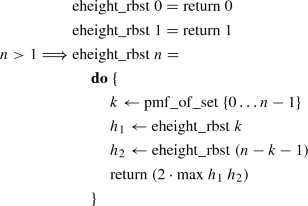Showing that this definition is indeed the correct one can be done by a straightforward induction following the recursive definition of $$\textit{rbst}$$:

### Lemma 4

(Correctness of $$\textit{eheight}\_\textit{rbst}$$)

\begin{aligned} \text {finite} \ A \Longrightarrow \mathrm{eheight\_rbst}\ |A| = \mathrm{map\_pmf}\ \text {eheight} \ (\mathrm{rbst}\ A) \end{aligned}

Using this, we note that for any $$n > 1$$:

\begin{aligned} \mathrm{E}[\widetilde{H}(n)]&= \frac{2}{n} \sum _{k=0}^{n-1} \mathrm{E}[\text {max} (\widetilde{H}(k), \widetilde{H}(n-k-1))]\\&\le \frac{2}{n} \sum _{k=0}^{n-1} \mathrm{E}[\widetilde{H}(k) + \widetilde{H}(n-k-1)]\\&= \frac{2}{n} \left( \sum _{k=0}^{n-1} \mathrm{E}[\widetilde{H}(k)] + \sum _{k=0}^{n-1} \mathrm{E}[\widetilde{H}(n-k-1)] \right) = \frac{4}{n} \sum _{k=0}^{n-1} \mathrm{E}[\widetilde{H}(k)] \end{aligned}

However, we still have to find a suitable polynomial upper bound to complete the induction argument. If we had some polynomial P(n) that fulfils $$0 \le P(0)$$, $$1 \le P(1)$$, and the recurrence $$P(n) = \frac{4}{n} \sum _{k=0}^{n-1} P(k)$$ , the above recursive estimate for $$\mathrm{E}[\widetilde{H}(n)]$$ would directly imply $$\mathrm{E}[\widetilde{H}(n)] \le P(n)$$ by induction. Cormen et al. give the following polynomial, which satisfies all these conditions and makes everything work out nicely:

\begin{aligned} P(n) = \frac{1}{4} {{n + 3} \atopwithdelims ()3} = \frac{1}{24} (n+1)(n+2)(n+3) \end{aligned}

Putting all of these together gives us the following theorem:

### Theorem 4

(Asymptotic expectation of H(n))

\begin{aligned} \mathrm{E}[H(n)]\ \le \ \log _2\mathrm{E}[\widetilde{H}(n)] + 1 \ \le \ \log _2 P(n) + 1\ \sim \ \frac{3}{\ln 2} \ln n \end{aligned}

## Treaps

As we have seen, BSTs have the nice property that even without any explicit balancing, they tend to be fairly balanced if elements are inserted into them in random order. However, if, for example, the elements are instead inserted in ascending order, the tree degenerates into a list and no longer has logarithmic height. One interesting way to prevent this is to use a randomised treap instead, which we shall introduce and analyse now.

### Definition

A treap is a binary tree in which every node contains both a ‘payload’ element and an associated priority (which, in our case, will always be a real number). A treap must be a BST w. r. t. the ‘payload’ elements and a heap w. r. t. the priorities (i. e. the root of any subtree is always a node with minimal priority in that subtree). This kind of structure was first described by Vuillemin  (who called it a Cartesian tree) and independently studied further by Seidel and Aragon , who noticed its relationship to random BSTs. Due to space constraints, we shall not go into how insertion of elements (denoted by $$\textit{ins}$$) works, but it is fairly easy to implement.

An interesting consequence of these treap conditions is that, as long as all of the priorities are distinct, the shape of a treap is uniquely determined by the set of its elements and their priorities. Since the sub-trees of a treap must also be treaps, this uniqueness property follows by induction and we can construct this unique treap for a given set using the following simple algorithm:

### Lemma 5

(Constructing the unique treap for a set) Let A be a set of pairs of type $$\alpha \times \mathbb {R}$$ where the second components are all distinct. Then there exists a unique treap $${\mathrm{treap}\_\mathrm{of}}\ A$$ whose elements are precisely A, and it satisfies the recurrence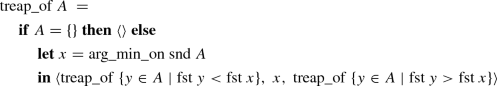where $$\mathrm{arg\_min\_on}\ f\ A$$ is some $$a \in A$$ such that f(a) is minimal on A. In our case the choice of a is unique by assumption.

This is very similar to the recurrence for bst_of_list that we saw earlier. In fact, it is easy to prove that if we forget about the priorities in the treap and consider it as a simple BST, the resulting tree is exactly the same as if we had first sorted the keys by increasing priority and then inserted them into an empty BST in that order. Formally, we have the following lemma:

### Lemma 6

(Connection between treaps and BSTs) Let p be an injective function that associates a priority to each element of a list $$\textit{xs}$$. Then

\begin{aligned}&\mathrm{map\_tree}\ \text {fst} \ (\mathrm{treap\_of}\ \{(x, p(x)) \mid x \in \text {set} \ \textit{xs}\}) = \mathrm{bst\_of\_list}\ (\mathrm{sort\_key}\ p\ \textit{xs})\,, \end{aligned}

where $$\textit{sort\_key}$$ sorts a list in ascending order w. r. t. the given priority function.

### Proof

By induction over $$\textit{xs}':= { sort\_key}\ p\ \textit{xs}$$, using the fact that sorting w. r. t. distinct priorities can be seen as a selection sort: The list $$\textit{xs}'$$ consists of the unique minimum-priority element x, followed by $${ sort\_key}\ p\ (\textit{remove1}\ x\ \textit{xs})$$, where $$\textit{remove1}$$ deletes the first occurrence of an element from a list.

With this and Lemma 2, the recursion structure of the right-hand side is exactly the same as that of the $${ treap\_of}$$ function from Lemma 5. $$\square$$

This essentially allows us to build a BST that behaves as if we inserted the elements by ascending priority regardless of the order in which they were actually inserted. In particular, we can assign each element a random priority upon its insertion, which turns our treap (a deterministic data structure for values of type $$\alpha \times \mathbb {R}$$) into a randomised treap, which is a randomised data structure for values of type $$\alpha$$ that has the same distribution (modulo the priorities) as a random BST with the same content.

One caveat is that for all the results so far, we assumed that no two distinct elements have the same priority, and, of course, without that assumption, we lose all these nice properties. If the priorities in our randomised treap are chosen from some discrete probability distribution, there will always be some non-zero probability that they are not distinct. For this reason, treaps are usually described in the literature as using a continuous distribution (e. g. uniformly-distributed real numbers between 0 and 1), even though this cannot be implemented faithfully on an actual computer. We shall do the same here, since it makes the analysis much easier.Footnote 1

The argument goes as follows:

1. 1.

Choosing the priority of each element randomly when we insert it is the same as choosing all the priorities beforehand (i. i. d. at random) and then inserting the elements into the treap deterministically.

2. 2.

By the theorems above, this is the same as choosing the priorities i. i. d. at random, sorting the elements by increasing priority, and then inserting them into a BST in that order.

3. 3.

By symmetry considerations, choosing priorities i. i. d. for all elements and then looking at the linear ordering defined by these priorities is the same as choosing one of the n! possible linear orderings uniformly at random.

4. 4.

Sorting a list according to a uniformly random linear ordering is the same as choosing a random permutation of the list uniformly at random.

5. 5.

Thus, inserting a list of elements into a randomised treap is the same as inserting them into a BST in random order.

### The Measurable Space of Trees

One complication when formalising treaps that is typically not addressed in pen-and-paper accounts is that since we will randomise over priorities, we need to talk about continuous distributions of trees, i. e. distributions of type $$(\alpha \times \mathbb {R})\ \textit{tree}\ \textit{measure}$$. For example, if we insert the element x into an empty treap with a priority that is uniformly distributed between 0 and 1, we get a distribution of trees with the shape $$\langle \langle \rangle , (x, p), \langle \rangle \rangle$$ where p is uniformly distributed between 0 and 1.

In order to be able to express this formally, we need a way to lift some measurable space M to a measurable space $$\mathcal {T}(M)$$ of trees with elements from M attached to their nodes. Formally, this $$\mathcal {T}$$ is an endofunctor in the category of measurable spaces; it maps a measurable space of elements to a corresponding measurable space of binary trees over those elements. Of course, we cannot just pick any measurable space: for our treap operations to be well-defined, all the basic tree operations need to be measurable w. r. t. $$\mathcal {T}(M)$$; in particular:

• the constructors $$\textit{Leaf}$$ and $$\textit{Node}$$, i. e. we need $$\{\textit{Leaf}\,\} \in \mathcal {T}(M)$$ and $$\textit{Node}$$ must be $$\mathcal {T}(M)\otimes M\otimes \mathcal {T}(M)$$$$\mathcal {T}(M)$$-measurable

• the projection functions (selecting the value / left sub-tree / right sub-tree of a node) must be measurable; e. g. selecting a node’s value must be $$(\mathcal {T}(M)\setminus \{\textit{Leaf}\,\})$$M-measurable

• primitively recursive functions involving only measurable operations must also be measurable; we will need this, for example, to show that the treap insertion operation is $$M\otimes \mathcal {B}\otimes \mathcal {T}(M\otimes \mathcal {B})$$$$\mathcal {T}(M\otimes \mathcal {B})$$-measurable (where $$\mathcal {B}$$ is the Borel $$\sigma$$-algebra of $$\mathbb {R}$$).Footnote 2

We can construct such a measurable space by taking the $$\sigma$$-algebra that is generated by certain cylinder sets: consider a tree whose nodes each have an M-measurable set attached to them. Then this tree can be ‘flattened’ into the set of trees of the same shape where each node has a single value from the corresponding set in t attached to it. Then we define $$\mathcal {T}(M)$$ to be the measurable space generated by all these cylinder sets.

To make this more formal, consider the following definitions in Isabelle:

\begin{aligned}&\mathrm{trees\_cyl} \,{:}{:}\, \alpha \ \mathrm{set\ tree} \rightarrow \alpha \ \mathrm{tree\ set}\\&\mathrm{trees\_cyl}\ \langle \rangle = \{\langle \rangle \}\\&\mathrm{trees\_cyl}\ \langle L, X, R\rangle = \bigcup \limits _{\ l\in \mathrm{trees\_cyl}\ L\ } \bigcup \limits _{x\in X}\bigcup \limits _{\ r\in \mathrm{trees\_cyl}\ R\ } \{\langle l, x, r\rangle \}\\&\mathrm{tree\_sigma} \,{:}{:}\, \alpha \ \text {measure} \rightarrow \alpha \ \mathrm{tree\ measure}\\&\mathrm{tree\_sigma}\ M = \text {sigma} \ (\text {trees} \ (\text {space} \ M))\ \{\mathrm{trees\_cyl}\ t\mid t \in \text {trees} \ (\text {sets} \ M)\} \end{aligned}

The function $$\textit{trees}\ A$$ simply returns the set of all trees where each node is restricted to having elements from A. The functions $$\textit{space}$$ and $$\textit{sets}$$ return the carrier set and the $$\sigma$$-algebra of a given measurable space. The function $$\textit{sigma}\ A\ \varSigma$$ constructs a measurable space with the given carrier set A and the $$\sigma$$-algebra generated by the set $$\varSigma$$.Footnote 3

The function trees_cyl constructs a cylinder set; it performs the above-mentioned ‘flattening’.Footnote 4 The function trees_sigma then corresponds to the functor $$\mathcal {T}$$; it simply constructs the $$\sigma$$-algebra generated by all the cylinder sets.

Given these definitions, proving that all the above-mentioned operations are indeed measurable is then straightforward, albeit somewhat tedious.

### Randomisation

Having taken care of the measure-theoretic background, we can now talk about randomised treaps. In order to achieve a good height distribution on average, the priorities of a treap must be random and independent of one another. In practice, we do not know how many elements will be inserted into the tree in advance, so we need to draw the priority to assign to an element when we insert it. Consequently, insertion is now a randomised operation.

### Definition 7

(Randomised insertion into a treap)

\begin{aligned}&\text {rins} \,{:}{:}\, \alpha \rightarrow \alpha \ \text {treap} \rightarrow \alpha \ \text {treap} \ \text {measure} \\&\text {rins} \ x\ t = \mathbf{do} \ \{p \leftarrow \mathrm{uniform\_measure}\ \{0\ldots {}1\};\ \text {return} \ (\text {ins} \ x\ p\ t) \} \end{aligned}

Since we would like to analyse what happens when we insert a large number of elements into an initially empty treap, we also define the following ‘bulk insert’ operation that inserts a list of elements into a treap from left to right:

\begin{aligned}&\text {rinss} \,{:}{:}\, \alpha \ \text {list} \rightarrow \alpha \ \text {treap} \ \rightarrow \alpha \ \text {treap} \ \text {measure} \\&\text {rinss} \ []\ t = \text {return} \ t\\&\text {rinss} \ (x\,\#\,\textit{xs})\ t = \mathbf{do} \ \{t' \leftarrow \text {rins} \ x\ t;\ \text {rinss} \ \textit{xs}\ t'\} \end{aligned}

Note that, from now on, we will again assume that all of the elements that we insert are distinct. This is not really a restriction, since inserting an element a second time does not change the tree at all, so we can just drop any duplicates from the list without changing the result. Similarly, the uniqueness property of treaps means that after deleting an element, the resulting treap is exactly the same as if the element had never been inserted in the first place, so even though we only analyse the case of insertions without duplicates, this extends to any sequence of insertion and deletion operations (although we do not show this explicitly).

The main result shall be that after inserting a certain number of distinct elements into the treap and then forgetting their priorities, we get a BST that is distributed identically to a random BST with the same elements, i. e. the treap behaves as if we had inserted the elements in random order. Formally, this can be expressed like this:

### Theorem 5

(Connecting randomised treaps to random BSTs)

\begin{aligned} \text {distr} \ (\text {rinss} \ \textit{xs}\ \langle \rangle )\ (\mathrm{map\_tree}\ \text {fst} ) = \mathrm{rbst}\ (\text {set} \ \textit{xs}) \end{aligned}

### Proof

Let $$\mathcal {U}$$ denote the uniform distribution of real numbers between 0 and 1 and $$\mathcal {U}^A$$ denote a vector of i. i. d. distributions $$\mathcal {U}$$, indexed by A:

\begin{aligned} \mathcal {U} := \mathrm{uniform\_measure}\ \{0\ldots {}1\} \quad \quad \mathcal {U}^A := \bigotimes \nolimits _{ A} \mathcal {U} \end{aligned}

The first step is to show that our bulk-insertion operation $$\textit{rinss}$$ is equivalent to first choosing random priorities for all the elements at once and then inserting them all (with their respective priorities) deterministically:

\begin{aligned} \text {rinss} \ \textit{xs}\ t&= \text {distr} \ \,\mathcal {U}^{\,\text {set} \,\textit{xs}}\ (\uplambda p.\ \text {foldl} \ (\uplambda t\ x.\ \text {ins} \ x\ (p(x))\ t)\ t\ \textit{xs})\\&= \text {distr} \ \,\mathcal {U}^{\,\text {set} \,\textit{xs}}\ (\uplambda p.\ \mathrm{treap\_of}\ [(x, p(x)) \mid x\leftarrow \textit{xs}]) \end{aligned}

The first equality is proved by induction over $$\textit{xs}$$, pulling out one insertion in the induction step and moving the choice of the priority to the front. This is intuitively obvious, but the formal proof is nonetheless rather tedious, mostly because of the issue of having to prove measurability in every single step. The second equality follows from the uniqueness of treaps.

Next, we note that the priority function returned by $$\mathcal {U}^{\,\text {set} \,\textit{xs}}$$ is almost surely injective, so we can apply Lemma 6 and get, all in all: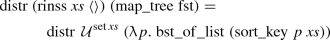The next key lemma is the following, which holds for any finite set A:

\begin{aligned} \text {distr} \ \,\mathcal {U}^A\ (\mathrm{linorder\_from\_keys}\ A) = \mathrm{uniform\_measure}\ (\mathrm{linorders\_on}\ A) \end{aligned}

This essentially says that choosing priorities for all elements of A and then looking at the ordering on A that these priorities induce will give us the uniform distribution on all the |A|! possible linear ordering relations on A. In particular, this means that the resulting order will be linear with probability 1, i. e. the priorities will almost surely be injective. The proof of this is a simple symmetry argument: given any two linear orderings R and $$R'$$ of A, we can find some permutation $$\pi$$ of A that maps $$R'$$ to R. However, $$\mathcal {U}^A$$ is stable under permutation. Therefore, R and $$R'$$ have the same probability, and since this holds for all R, $$R'$$, the distribution must be the uniform distribution.

This brings us to the last step: Proving that sorting our list of elements by random priorities and then inserting them into a BST is the same as inserting them in random order (in the sense of inserting them in the order given by a randomly-permuted list):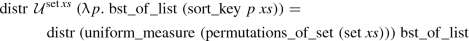Here we use the fact that priorities chosen uniformly at random induce a uniformly random linear ordering, and that sorting a list with such an ordering produces permutations of that list uniformly at random. The proof of this involves little more than rearranging and using some obvious lemmas on $$\textit{sort\_key}$$ etc. Now the right-hand side is exactly the definition of a random BST (up to a conversion between $$\textit{pmf}$$ and $$\textit{measure}$$), which concludes the proof. $$\square$$

In the real world, generating ideal real numbers at random as we assumed is, of course, impractical. Another, simpler solution would be to use integers from a finite range as priorities. This is not a faithful implementation, but Seidel and Aragon themselves already provided an analysis of this in which they prove that if the integer range is reasonably large, the randomised treaps still work as intended except for a small probability even if the priorities are not fully independent. This analysis could probably be added to our formalisation with a moderate amount of effort; however, we considered this to be out of scope for this work.

## Randomised BSTs

Another approach to use randomisation in order to obtain a tree data structure that behaves essentially like a random BST irrespectively of the order of insertion are the Randomised BSTs introduced by Martínez and Roura . We will call them MR trees from now on. The key difference between randomised treaps and MR trees is the following:

In treaps, the randomness lies in the priorities associated with each entry. This priority is chosen once for each key and never modified. The algorithms that operate on the tree itself (insertion, deletion, etc.) are completely deterministic; their randomness comes only from the random priorities that were chosen in advance.

In MR trees, on the other hand, there is no extra information associated to the nodes. The randomness is introduced through coin flips in every recursive step of the tree operations (insertion, deletion, etc). Unlike with treaps, the results of these random choices are not stored in the tree at all (although they do, of course, influence the structure of the resulting tree). Another important difference is that, seeing as they are coin flips, the random choices that are made are discrete.

Note that while we said above that MR trees do not contain any additional new information, it is necessary to add cached information for an efficient implementation: the precise coin weights at each step depend on the total number of nodes in the current sub-tree. Since this number takes linear time to compute and we are aiming for logarithmic time, it needs to be cached in each node. However, unlike with treaps, this can be ignored completely in the correctness analysis of the algorithm. It could easily be added in a refinement step later on.

On an abstract level, we can therefore simply see MR trees as normal BSTs. All operations on MR trees work correctly on any BST, but they additionally have the property that if the input trees are random BSTs, the output tree is also a random BST. This gives us two correctness properties for each operation: a deterministic one and a probabilistic one. The former is trivial to show in all cases, whereas the latter usually requires some lengthy (but fairly straightforward) manipulations in the Giry monad. Each probabilistic theorem actually implies its deterministic counterpart since the support of random_bst is the set of all BSTs, but it is convenient to already have access to the deterministic version when proving the probabilistic one and the deterministic ones are very easy to prove. We therefore always first proved the deterministic ones and then the probabilistic ones.

Let us now first look at the implementation of the operations themselves. For a more didactic and informal introduction, we refer the reader to the original presentation by Martínez and Roura . While Martínez and Roura present the algorithms in a C-like imperative style, we will again show them in a functional Giry-monad presentation that is very close to the definitions in Isabelle.

### Auxiliary Operations

Following Martínez and Roura, we first need to define three auxiliary functions to split and join BSTs.

#### Splitting

The first function splits a BST into two halves w. r. t. a key x that may or may not be in the tree:

### Definition 8

(Splitting a BST)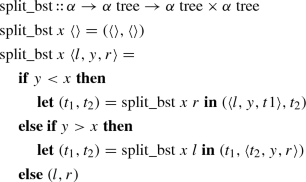### Theorem 6

(Correctness of Splitting) The deterministic correctness property here simply means that if this function is applied to a BST t, it returns a pair of BSTs (lr) consisting of all the elements that are strictly smaller (resp. strictly greater) than x. In Isabelle notation:

\begin{aligned}&\text {bst} \ l\ \wedge \ \mathrm{set\_tree}\ l = \{y\in \mathrm{set\_tree}\ t \mid y < x\}\ \wedge {}\\&\text {bst} \ r\ \wedge \ \mathrm{set\_tree}\ r = \{y\in \mathrm{set\_tree}\ t \mid y > x\} \end{aligned}

The probabilistic correctness property states that if the function is applied to a random BST, it will again return a pair of two independent random BSTs: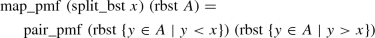#### Joining

Next, we define a kind of inverse operation for $$\textit{split}\_\textit{bst}$$ that computes the union of two BSTs $$t_1$$ and $$t_2$$ under the precondition that all values in $$t_1$$ are strictly smaller than those in $$t_2$$. The idea is essentially to build up the tree top-down, tossing a weighted coin in each step to determine whether to insert a branch from $$t_1$$ or from $$t_2$$ as the next element:

### Definition 9

(Joining two MR trees)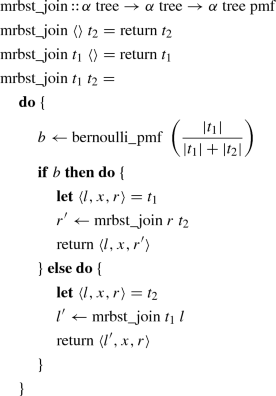### Theorem 7

(Correctness of Joining) The deterministic correctness theorem for $$\textit{mrbst}\_\textit{join}$$ is the following: Let $$t_1$$ and $$t_2$$ be BSTs such that all elements in $$t_1$$ are strictly smaller than all elements in $$t_2$$. Furthermore, let t be a tree in the support of $$\mathrm{mrbst\_join}\ t_1\ t_2$$. Then:

\begin{aligned} \text {bst} \ t\ \ \wedge \ \ \mathrm{set\_tree}\ t = \mathrm{set\_tree}\ t_1 \cup \mathrm{set\_tree}\ t_2 \end{aligned}

As for the probabilistic theorem: Let A and B be two finite sets with $$\forall x{\in }A, y{\in }B.\ x < y$$. Then:

\begin{aligned} \mathbf{do} \ \{t_1\leftarrow \mathrm{rbst}\ A;\ t_2\leftarrow \mathrm{rbst}\ B;\ \mathrm{mrbst\_join}\ t_1\ t_2\}\ =\ \mathrm{rbst}\ (A\cup B) \end{aligned}

From now on, we will not print the deterministic correctness theorems anymore since they are always analogous to the probabilistic ones and not very interesting.

#### Pushdown

The function $${ mrbst\_join}$$ is an inverse operation to $${ split\_bst}\ x$$ if x is not present in the tree. However, we will also need an inverse operation for the case that xis present in the tree. Following Martínez and Roura, this operation is called $${ mrbst\_push\_down}\ l\ x\ r$$. The situation where it is used can also be thought of like this: as we have noted before (in Lemma 3), the distribution $$\textit{rbst}\ A$$ for a non-empty set A can be decomposed like this:

\begin{aligned} \mathbf{do} \ \{&x\leftarrow \mathrm{pmf\_of\_set}\ A;\ l\leftarrow \mathrm{rbst}\ \{y\in A\mid y < x\};\nonumber \\ {}&r\leftarrow \mathrm{rbst}\ \{y\in A\mid y > x\};\ \text {return} \ \langle l, x, r\rangle \} \end{aligned}
(2)

Now suppose we do not choose x at random, but rather do the above for a fixed $$x\in A$$, i. e. we know what the root is, but the rest is random:

\begin{aligned} \mathbf{do} \ \{l\leftarrow \mathrm{rbst}\ \{y\in A\mid y < x\};\ r\leftarrow \mathrm{rbst}\ \{y\in A\mid y > x\};\ \text {return} \ \langle l, x, r\rangle \} \end{aligned}
(3)

The purpose of $${ mrbst\_push\_down}$$ is then to transform the ‘almost random BST’ distribution described by (3) into the ‘proper random BST’ distribution described by (2). In a sense, $${ mrbst\_push\_down}$$ allows us to forget what the root is.

The definition of $${ mrbst\_push\_down}$$ is similar to $${ mrbst\_join}$$ except that we now have a three-way split: In every step, we toss a weighted ‘three-sided coin’ to determine whether to insert a branch from l, a branch from r, or x itself (which stops the recursion):

### Definition 10

(Pushdown of MR trees)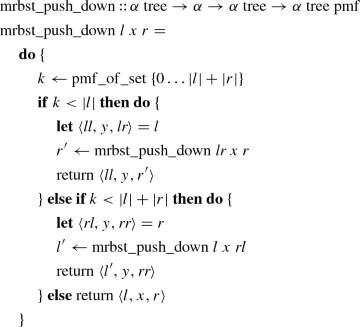### Theorem 8

(Correctness of Pushdown) Let x be an element and A and B be finite sets with $$\forall y{\in }A.\ y < x$$ and $$\forall y{\in }B.\ y > x$$. Then:

\begin{aligned} \mathbf{do} \ \{l\leftarrow \mathrm{rbst}\ A;\ r\leftarrow \mathrm{rbst}\ B;\ \mathrm{mrbst\_push\_down}\ l\ x\ r\}\ =\ \mathrm{rbst}\ (\{x\}\cup A\cup B) \end{aligned}

### Main Operations

We now turn towards the main operations: insertion, deletion, union, intersection, and difference. We begin with the last two.

#### Intersection and Difference

Unlike Martínez & Roura, we have unified intersection and difference of two MR trees into a single algorithm in order to avoid a duplication of proofs. The definition is fairly simple and uses the auxiliary operations we have defined so far:Footnote 5

### Definition 11

(Intersection and Difference of MR Trees)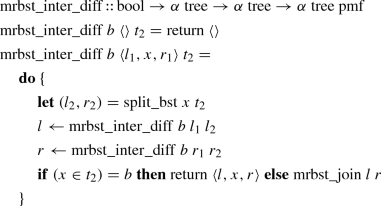Choosing $$b := \text {True}$$ yields the intersection of $$t_1$$ and $$t_2$$; choosing $$b := \text {False}$$ yields their difference.

### Theorem 9

(Correctness of Intersection and Difference)

\begin{aligned}&\mathbf{do} \ \{t_1\leftarrow \mathrm{rbst}\ A;\ t_2\leftarrow \mathrm{rbst}\ B;\ \mathrm{mrbst\_inter\_diff}\ \text {True} \ t_1\ t_2\} = \mathrm{rbst}\ (A \cap B)\\&\mathbf{do} \ \{t_1\leftarrow \mathrm{rbst}\ A;\ t_2\leftarrow \mathrm{rbst}\ B;\ \mathrm{mrbst\_inter\_diff}\ \text {False} \ t_1\ t_2\} = \mathrm{rbst}\ (A \setminus B) \end{aligned}

#### Union

The union of two MR trees is somewhat trickier to define:

### Definition 12

(Union of MR trees)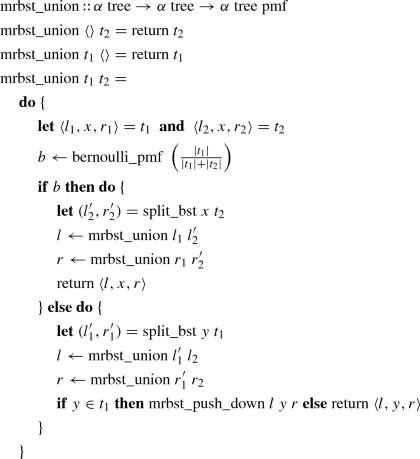### Theorem 10

(Correctness of Union)

\begin{aligned}&\mathbf{do} \ \{t_1\leftarrow \mathrm{rbst}\ A;\ t_2\leftarrow \mathrm{rbst}\ B;\ \mathrm{mrbst\_union}\ t_1\ t_2\} = \mathrm{rbst}\ (A \cup B) \end{aligned}

#### Derived Operations

We omit the definitions of the insertion and deletion algorithms here since they are just specialised and ‘inlined’ versions of the union and difference algorithms with the first (resp. second) input taken to be a singleton tree $$\langle \langle \rangle , x, \langle \rangle \rangle$$. This is also how the correctness of insertion and deletion was shown in Isabelle: The algorithms were defined recursively just like given by Roura & Martínez. Then, we show

\begin{aligned} \mathrm{mrbst\_insert\ x\ t}&= \mathrm{mrbst\_union}\ \langle \langle \rangle , x, \langle \rangle \rangle \ t\\ \mathrm{mrbst\_delete\ x\ t}&= \mathrm{mrbst\_inter\_diff}\ \text {False} \ t\ \langle \langle \rangle , x, \langle \rangle \rangle \end{aligned}

by a straightforward (and fully automatic) induction, so that the correctness results for $${ mrbst\_union}$$ and $${ mrbst\_inter\_diff}$$ carry over directly.

### Proofs

The correctness proofs are all fairly straightforward and—save for one exception that we will discuss later—purely compositional. By compositional, we mean that one simply rearranges and rewrites parts of the expression on the left-hand side until one reaches the right-hand side, as opposed to proving equality by a ‘brute force’ computation of the probabilities. This compositional nature makes the proofs much easier to follow. Indeed, although the declarative nature of Isabelle’s proof language Isar and the large sub-expressions that occur make them rather large, the Isabelle proofs are fairly readable and comparable to a detailed pen-and-paper proof of the same statement.

The key ingredients in the proofs are again the recurrence equation for $$\textit{rbst}$$ (Lemma 3) and the fact that choosing uniformly at random from a disjoint union $$A\cup B$$ can be replaced by tossing a coin weighted with $$\frac{|A|}{|A|+|B|}$$ and then choosing uniformly at random either from A (for heads) or from B (for tails):

### Lemma 7

Let $$A\cap B = \emptyset$$, $$A \cup B\ne \emptyset$$, and define $$p := \frac{|A|}{|A| + |B|}$$. Then:

\begin{aligned} \mathrm{pmf\_of\_set}\ (A\cup B) = \mathbf{do} \ \{&b\leftarrow \mathrm{bernoulli\_pmf}\ p;\\&\mathbf{if} \ b\ \mathbf{then} \ \mathrm{pmf\_of\_set}\ A\ \mathbf{else} \ \mathrm{pmf\_of\_set}\ B\} \end{aligned}

### Proof

By extensionality (i. e. computing the probabilities of each side). $$\square$$

Similarly, a uniform random choice from a disjoint union of three sets A, B, and C can be replaced by choosing a number k between 0 and $$|A|+|B|+|C|-1$$ and then distinguishing the three cases $$k < |A|$$ , $$|A| \le k < |A| + |B|$$ , and $$k \ge |A| + |B|$$.

Apart from this, the proofs are mostly a matter of rearranging in the Giry monad using the basic monad laws in addition to commutativity

\begin{aligned}&\mathbf{do} \ \{x\leftarrow A;\ y\leftarrow B;\ C\ x\ y\} = \mathbf{do} \ \{y\leftarrow B;\ x\leftarrow A;\ C\ x\ y\} \end{aligned}

and the absorption property

\begin{aligned}&\mathbf{do} \ \{x\leftarrow A;\ B\} = B \end{aligned}

where A does not depend on y and B does not depend on x.

Martínez and Roura actually gave some sketches of formal correctness proofs, using an algebraic notation for probabilistic programs that they developed (they were apparently not aware of the Giry monad). Their notation is very similar to the Giry monad, except that no distinction is made between deterministic and randomised operations and the monadic bind and return are fully implicit. They give proof sketches for some of the MR tree operations, but parts of them are rather vague. It seems that their intent was more to use the notation to state their correctness theorems in a rigorous formal way than to give rigorous formal proofs.

Notational differences aside, the main other difference to our proofs is that they shift most of their reasoning from the realm of random BSTs to that of random permutations. It seems that their approach works as well; we do not know if either way is significantly more or less difficult, but we suspect it does not make much of a difference.

#### Correctness Proof for ‘Join’

As a representative example, we will show the proof for the joining operation here. The other proofs all follow a very similar approach and are very straightforward.

### Proof

The proof is an induction following the recursive definition of $${ mrbst\_join}$$. The base cases are trivial; in the induction step, we have to show

\begin{aligned} \mathrm{rbst}\ (A \cup B) = \mathbf{do} \ \{t_1 \leftarrow \mathrm{rbst}\ A;\ t_2 \leftarrow \mathrm{rbst}\ B;\ \mathrm{mrbst\_join}\ t_1\ t_2\} \end{aligned}

for non-empty sets A and B where all elements of A are strictly smaller than all elements of B. We will show this by successively rewriting the right-hand side: Let us define $$p := \frac{|A|}{|A| + |B|}$$ and note that $$|t_1| = |A|$$ and $$|t_2| = |B|$$. We then unfold one step of the definition of $$mrbst\_join$$ (see Definition 9) and note that the right-hand side simplifies to: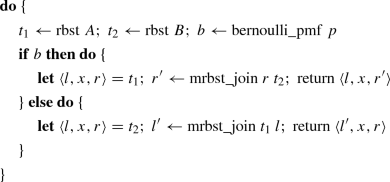Using distributivity of if and commutativity, we obtain: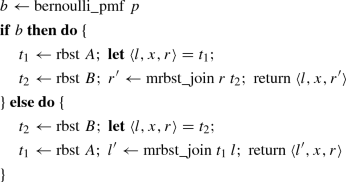Using the recurrence relation for $$\textit{rbst}$$ (Lemma 3), we obtain: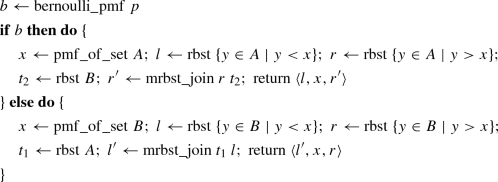Let us now focus on the ‘then’ branch. We can rearrange this to

\begin{aligned}&x \leftarrow \mathrm{pmf\_of\_set}\ A;\ l \leftarrow \mathrm{rbst}\ \{y\in A\mid y < x\};\\&r' \leftarrow \mathbf{do} \ \{r \leftarrow \mathrm{rbst}\ \{y\in A\mid y > x\};\ t_2\leftarrow \mathrm{rbst}\ B;\ r' \leftarrow \mathrm{mrbst\_join}\ r\ t_2\};\\&\text {return} \ \langle l, x, r'\rangle \end{aligned}

By induction hypothesis, the second line can be simplified to

\begin{aligned} r' \leftarrow \mathrm{rbst}\ (\{y\in A\mid y > x\} \cup B)\ . \end{aligned}

Moreover, since all elements of A are smaller than all elements in B by assumption, we have

\begin{aligned} \{y\in A \cup B\mid y< x\}&= \{y\in A\mid y < x\}\\ \{y\in A \cup B\mid y> x\}&= \{y\in A\mid y > x\} \cup B \end{aligned}

so that the ‘then’ branch is now:

\begin{aligned}&x \leftarrow \mathrm{pmf\_of\_set}\ A;\ l \leftarrow \mathrm{rbst}\ \{y\in A\cup B\mid y < x\};\ r \leftarrow \mathrm{rbst}\ \{y\in A\cup B\mid y > x\}\;\\&\text {return} \ \langle l, x, r\rangle \end{aligned}

With analogous reasoning for the ‘else’ branch, we get: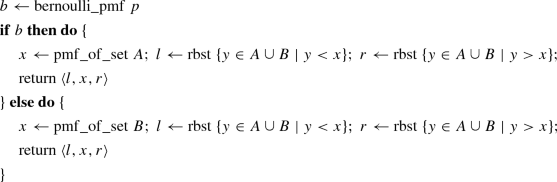The two branches now only differ in that the first one chooses $$x\leftarrow { pmf\_of\_set}\ A$$ while the second one chooses $$x\leftarrow { pmf\_of\_set}\ B$$. We rearrange using commutativity and distributivity of if to obtain:

\begin{aligned}&b \leftarrow \mathrm{bernoulli\_pmf}\ p;\ x \leftarrow (\mathbf{if} \ b\ \mathbf{then} \ \mathrm{pmf\_of\_set}\ A\ \mathbf{else} \ \mathrm{pmf\_of\_set}\ B);\\&l \leftarrow \mathrm{rbst}\ \{y\in A\cup B\mid y < x\};\ r \leftarrow \mathrm{rbst}\ \{y\in A\cup B\mid y > x\};\\&\text {return} \ \langle l, x, r\rangle \end{aligned}

Since b only occurs in the first line, we can use the monad laws and absorption to rewrite the first line to

\begin{aligned} x\leftarrow \mathbf{do} \ \{b\leftarrow \mathrm{bernoulli\_pmf}\ p;\ \mathbf{if} \ b\ \mathbf{then} \ \mathrm{pmf\_of\_set}\ A\ \mathbf{else} \ \mathrm{pmf\_of\_set}\ B\}\ , \end{aligned}

which, by Lemma 7, is equivalent to $$x\leftarrow { pmf\_of\_set}\ (A \cup B)$$ so that we now have: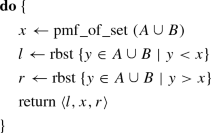By the recurrence equation for $$\textit{rbst}$$ (Lemma 3), this is simply $$\textit{rbst}\ (A\cup B)$$. $$\square$$

While the proofs for the other operations all differ from one another significantly, the general strategy is always the same:

1. 1.

push everything into the conditional (apart from the coin flip on which the conditional depends, of course)

2. 2.

rearrange all branches in order to apply the induction hypothesis

3. 3.

rearrange all branches so that they only differ in the first step

4. 4.

extract that first step and ‘merge’ it with the initial coin flip

#### Correctness Proof for ‘Union’

The only operation whose proof is slightly more complicated is the union operation. We will skip the beginning of the proof and delve straight into the difficult part: Recall Definition 12. Following the approach outlined above, we rewrite the right-hand side of the recursive case and eventually arrive at the following expression: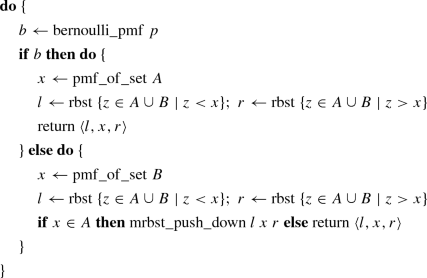In order to proceed, the case distinction over $$x\in A$$ must be eliminated. Since the ‘$$x\notin A$$’ case of the ‘else’ branch is identical to the ‘then’ branch, it seems a reasonable goal to merge these two. The route we followed for this was to again split the uniform choice $$x\leftarrow { pmf\_of\_set}\ B$$ with a coin flip followed by a uniform choice from $$A\cap B$$ resp. $$B\setminus A$$. The weight q of the coin flip must be $$|A \cap B| / |B|$$. We now have: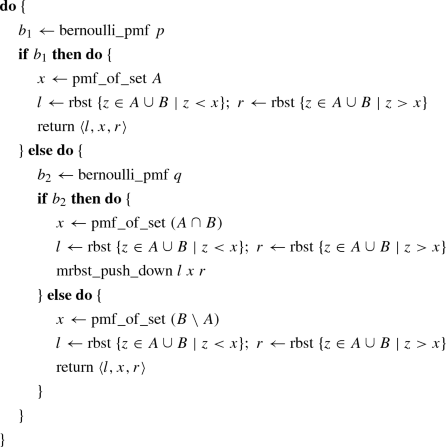After some rearrangement, we obtain: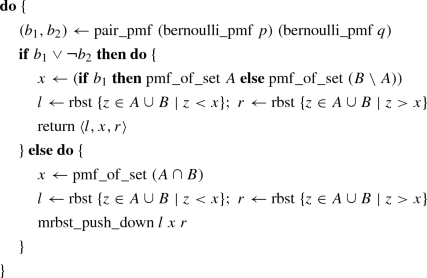Applying the correctness theorem for the pushdown operation and discarding the now unused bind $$x \leftarrow { pmf\_of\_set}\ (A\cap B)$$, the ‘$$\mathbf{else}$$’ branch simplifies to just $$\textit{rbst}\ (A \cup B)$$ as desired. To continue simplifying the other branch, we modify the pair of Booleans chosen in the first step by drawing them directly from the distribution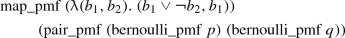which can be shown to be equal to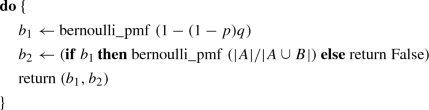by a simple ‘brute force’ extensionality argument. One could also make this argument by using conditional probability explicitly, but in this simple situation, the brute force argument is probably shorter.

We then have the expression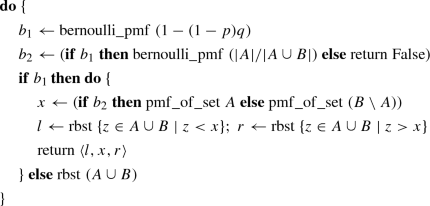which can be rearranged to: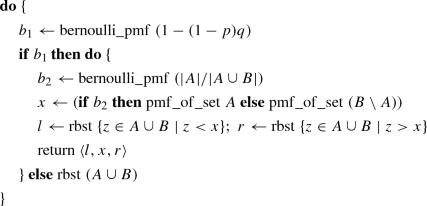Applying Lemma 7 to the disjoint union $$A \cup (B\setminus A)$$ yields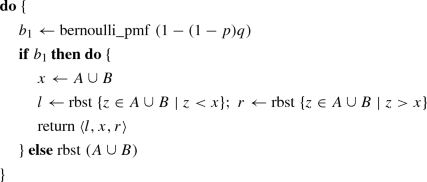and with a final application of the recurrence relation Lemma 3, the entire expression simplifies to $$\textit{rbst}\ (A \cup B)$$ as desired. $$\square$$

### Proof Automation

Due to the higher-order nature of the monadic bind operation, normalising such expressions is too difficult for Isabelle’s simplifier. Applying the simplifier naïvely can often lead to non-termination. We therefore used a proof method developed by Schneider et al.  that partially solves this problem. It works for PMFs only (since monadic operations on general measures cannot be rearranged so easily due to side conditions) and was not available when the work described in the first few sections was done, so we only used it for the proofs about MR trees. Unfortunately, their method is not complete (which they do mention in their article) and there were occasional situations in our proofs that actually exposed this incompleteness: It e. g. fails to prove the following statement (which is obviously true in a commutative monad) as it considers both sides to be in ‘normal form’:

\begin{aligned}&\mathbf{do} \ \{a \leftarrow f\ A;\ b \leftarrow f\ B;\ c \leftarrow D\ b;\ d \leftarrow f\ C;\ F\ a\ c\ d\} = {}\\&\mathbf{do} \ \{b \leftarrow f\ B;\ c \leftarrow D\ b;\ a \leftarrow f\ A;\ d \leftarrow f\ C;\ F\ a\ c\ d\} \end{aligned}

We believe that a more involved approach to normalise such expressions could potentially solve this problem. Let us briefly sketch this idea here: A monadic expression can be transformed into a kind of rooted ordered DAG where

• the nodes are the monadic computations (e. g. $$a \leftarrow f\ A$$ above)

• each node has one edge for each monadically bound variable that appears in it, ordered left-to-right (e. g. $$F\ a\ c\ d$$ would have three children: the nodes corresponding to $$a\leftarrow f\ A$$, $$c\leftarrow D\ b$$, etc.)

• the root is the ‘result’, i. e. the $$F\ a\ c\ d$$ above).

Then, a canonical reordering of the expression can be computed using a post-order traversal of the DAG.

‘Unused’ binds make the situation more difficult since they lead to additional roots without an obvious canonical ordering—however, this problem is only relevant for monads that do not have the previously-mentioned absorption property (e. g. monads that can ‘fail’, like the option and set monads). For the Giry monad, this particular problem does not occur.

A complication of practical importance that does occur, however, is dealing with control flow structures like case and if that distribute over monadic binds. It is not clear to us what a complete method that also incorporates these properties could look like.

## Related Work

The earliest analysis of randomised algorithms in a theorem prover was probably by Hurd  in the HOL system, who modelled them by assuming the existence of an infinite sequence of random bits which programs can consume. He used this approach to formalise the Miller–Rabin primality test.

Audebaud and Paulin-Mohring  created a shallowly-embedded formalisation of (discrete) randomised algorithms in Coq and demonstrated its usage on two examples. Barthe et al.  used this framework and their probabilistic relational Hoare logic (pRHL) to implement the CertiCrypt system to write machine-checked cryptographic proofs for a deeply embedded imperative language with ‘while’ loops. Petcher and Morrisett  developed a similar framework called FCF, which is instead based on a semi-shallow monadic embedding.

There are many similarities between these approaches and ours, but unlike them, our probabilistic expressions are written directly in the logic of Isabelle/HOL without any underlying notion of ‘program’ or ‘expression’. Consequently, we do not use any kind of program logic either. Moreover, in contrast to Barthe et al., we use recursion instead of loops—which is essential for algorithms such as QuickSort, which do not have a straightforward non-recursive formulation.

Lochbihler  developed another similar framework in Isabelle/HOL which is much closer to our approach. Like we, he uses PMFs directly in the logic without any embedding, although he also introduces some additional explicit embeddings that are specific to the domain of cryptography. He also provides a more in-depth comparison of the expressiveness of his approach and other systems like CertiCrypt and FCF that also applies to our work.

There are two major differences between these last three approaches and ours: First of all, all of them focus heavily on cryptographic algorithms and protocols. Second, they use relational reasoning. Although Isabelle’s measure theory library does support relational reasoning, we did not make any use of it since all of our reasoning can be done comfortably using only equality of distributions. This can, of course, be seen as relational reasoning as well, but we do not believe there is any benefit in doing so.

The expected running time of randomised quicksort (possibly including repeated elements) was first analysed in a theorem prover by van der Weegen and McKinna  using Coq. They proved the upper bound $$2n \lceil \log _2 n\rceil$$, whereas we actually proved the closed-form result $$2 (n + 1) H_n - 4n$$ and its precise asymptotics. Although their paper’s title mentions ‘average-case complexity’, they, in fact, only treat the expected running time of the randomised algorithm in their paper. They did, however, later add a separate proof of an upper bound for the average-case of deterministic quicksort to their GitHub repository. Unlike us, they allow lists to have repeated elements even in the average case, but they proved the expectation bounds separately and independently, while we assumed that there are no repeated elements, but showed something stronger, namely that the distributions are exactly the same, allowing us to reuse the results from the randomised case.

Kaminski et al.  presented a Hoare-style calculus for analysing the expected running time of imperative programs and used it to analyse a one-dimensional random walk and the Coupon Collector problem. Hölzl  formalised this approach in Isabelle and found a mistake in their proof of the random walk in the process.

At the same time as our work and independently, Tassarotti and Harper  gave a Coq formalisation of a cookbook-like theorem based on work by Karp  that is able to provide tail bounds for a certain class of randomised recurrences such as the number of comparisons in quicksort and the height of a random BST. In contrast to the expectation results we proved, such bounds are very difficult to obtain on a case-by-case basis, which makes such a cookbook-like result particularly useful.

Outside the world of theorem provers, other approaches exist for automating the analysis of such algorithms: Probabilistic model checkers like PRISM  can check safety properties and compute expectation bounds. The $$\Lambda \Upsilon \varOmega$$ system by Flajolet et al.  conducts a fully automatic analysis of average-case running time for a restricted variety of (deterministic) programs. Chatterjee et al.  developed a method for deriving bounds of the shape $$O(\ln n)$$, O(n), or $$O(n \ln n)$$ for certain recurrences that are relevant to average-case analysis automatically and applied it to a number of interesting examples, including quicksort.

## Conclusion

We have closed a number of important gaps in the formalisation of classic probabilistic algorithms related to binary search trees, including the thorny case of treaps, which requires measure theory. Up to that point we claim that these formalisations are readable (the definitions thanks to the Giry monad and the proofs thanks to Isar ), but for treaps this becomes debatable: the issue of measurability makes proofs and definitions significantly more cumbersome and less readable. Although existing automation for measurability is already very helpful, there is still room for improvement. Moreover, the construction of the measurable space of trees generalises to other data types and could be automated.

All of our work so far has been at the functional level, but it would be desirable to refine it to the imperative level in a modular way. The development of the necessary theory and infrastructure is future work.

1. In fact, any non-discrete probability distribution works, where by ‘non-discrete’ we mean that all singleton sets have probability 0. In the formalisation, however, we restricted ourselves to the case of a uniform distribution over a real interval.

2. We also need the ‘<’ operation on M to be measurable w. r. t. M for this to work. In our case, this will not be an issue, since we only have finitely many elements to insert into our tree so that we can choose the carrier of M to be finite. Then, we can always use the discrete (or power set) $$\sigma$$-algebra $$2^M$$, for which all functions are measurable.

3. Some readers may wonder why $${ tree\_sigma}$$ operates on the $$\textit{measure}$$ type – which we introduced before as the type for measure spaces—even though it abstractly operates on measurable spaces, not measure spaces. The reason for this is simply that, for convenience, Isabelle’s probability theory library identifies measurable spaces with measure spaces where the measure function is ignored.

4. Readers familiar with Haskell might find this easier to visualise by thinking of $$\mathrm{trees\_cyl}$$ as the $$\textit{sequence}$$ operation in the $$\textit{Set}$$ monad, i. e. $$\textit{sequence} \,{:}{:}\, \text {Tree} \ (\text {Set} \ a) \rightarrow \text {Set} \ (\text {Tree} \ a)$$ .

5. Note that for efficient implementation, the test $$x\in t_2$$ does not actually have to traverse the tree again; one can simply modify $$split\_bst$$ such that it also returns (at no extra cost) a Boolean indicating whether the key x was in the tree or not. This is also how it is defined in Isabelle.

## References

1. Aslam, J.A.: A simple bound on the expected height of a randomly built binary search tree. Technical Report TR2001-387, Dartmouth College, Hanover, NH (2001). Abstract and paper lost

2. Audebaud, P., Paulin-Mohring, C.: Proofs of randomized algorithms in Coq. Sci. Comput. Program. 74(8), 568–589 (2009). https://doi.org/10.1016/j.scico.2007.09.002

3. Barthe, G., Grégoire, B., Béguelin, S.Z.: Formal certification of code-based cryptographic proofs. In: Proceedings of the 36th ACM SIGPLAN-SIGACT Symposium on Principles of Programming Languages, POPL 2009, pp. 90–101 (2009). https://doi.org/10.1145/1480881.1480894

4. Basin, D.A., Lochbihler, A., Sefidgar, S.R.: CryptHOL: Game-based proofs in higher-order logic. Cryptology ePrint Archive, Report 2017/753 (2017). https://doi.org/10.1007/978-3-662-49498-1_20. https://eprint.iacr.org/2017/753

5. Chatterjee, K., Fu, H., Murhekar, A.: Automated recurrence analysis for almost-linear expected-runtime bounds. In: Computer Aided Verification: 29th International Conference, CAV 2017, pp. 118–139 (2017). https://doi.org/10.1007/978-3-319-63387-9_6

6. Cichoń, J.: Quick Sort: average complexity. http://cs.pwr.edu.pl/cichon/Math/QSortAvg.pdf Accessed 13 Mar 2017

7. Cormen, T.H., Stein, C., Rivest, R.L., Leiserson, C.E.: Introduction to Algorithms, 2nd edn. McGraw-Hill Higher Education, New York (2001)

8. Eberl, M.: Expected shape of random binary search trees. Archive of Formal Proofs (2017). http://isa-afp.org/entries/Random_BSTs.html, Formal proof development

9. Eberl, M.: The number of comparisons in QuickSort. Archive of Formal Proofs (2017). http://isa-afp.org/entries/Quick_Sort_Cost.html, Formal proof development

10. Eberl, M.: Randomised binary search trees. Archive of Formal Proofs (2018). http://isa-afp.org/entries/Randomised_BSTs.html, Formal proof development

11. Eberl, M., Haslbeck, M.W., Nipkow, T.: Verified analysis of random trees. In: Proceedings of the 9th International Conference on Interactive Theorem Proving (2018). https://doi.org/10.1007/978-3-319-94821-8

12. Eberl, M., Hölzl, J., Nipkow, T.: A verified compiler for probability density functions. In: J. Vitek (ed.) Proceedings of the 24th European Symposium on Programming, pp. 80–104. Springer, Berlin Heidelberg (2015). https://doi.org/10.1007/978-3-662-46669-8_4

13. Flajolet, P., Salvy, B., Zimmermann, P.: Lambda - Upsilon - Omega: An assistant algorithms analyzer. In: 6th International Conference Applied Algebra, Algebraic Algorithms and Error-Correcting Codes, AAECC-6, Rome, Italy, July 4–8, 1988, Proceedings, pp. 201–212 (1988). https://doi.org/10.1007/3-540-51083-4_60

14. Giry, M.: A categorical approach to probability theory. In: Categorical Aspects of Topology and Analysis, Lecture Notes in Mathematics, vol. 915, pp. 68–85. Springer Berlin (1982). https://doi.org/10.1007/BFb0092872

15. Gouëzel, S.: Ergodic theory. Archive of Formal Proofs (2015). http://isa-afp.org/entries/Ergodic_Theory.html, Formal proof development

16. Haslbeck, M., Eberl, M., Nipkow, T.: Treaps. Archive of Formal Proofs (2018). http://isa-afp.org/entries/Treaps.html, Formal proof development

17. Hoare, C.A.R.: Quicksort. Comput. J. 5(1), 10 (1962). https://doi.org/10.1093/comjnl/5.1.10

18. Hölzl, J.: Formalising semantics for expected running time of probabilistic programs. In: J.C. Blanchette, S. Merz (eds.) Interactive Theorem Proving (ITP 2016), pp. 475–482. Springer, Berlin (2016). https://doi.org/10.1007/978-3-319-43144-4_30

19. Hölzl, J.: Markov chains and Markov decision processes in Isabelle/HOL. J. Autom. Reason. (2017). https://doi.org/10.1007/s10817-016-9401-5

20. Hölzl, J., Heller, A.: Three chapters of measure theory in Isabelle/HOL. In: Interactive Theorem Proving—Second International Conference, ITP 2011, Berg en Dal, The Netherlands, August 22–25, 2011. Proceedings, pp. 135–151 (2011). https://doi.org/10.1007/978-3-642-22863-6_12

21. Hurd, J.: Formal verification of probabilistic algorithms. Ph.D. thesis, University of Cambridge (2002)

22. Kaminski, B.L., Katoen, J.P., Matheja, C., Olmedo, F.: Weakest precondition reasoning for expected run—times of probabilistic programs. In: Proceedings of the 25th European Symposium on Programming Languages and Systems: volume 9632, pp. 364–389. Springer-Verlag New York, Inc., New York, NY, USA (2016). https://doi.org/10.1007/978-3-662-49498-1_15

23. Karp, R.M.: Probabilistic recurrence relations. J. ACM 41(6), 1136–1150 (1994). https://doi.org/10.1145/195613.195632

24. Knuth, D.E.: The Art of Computer Programming, Volume 3: Sorting and Searching. Addison Wesley Longman Publishing Co., Inc., Redwood City (1998)

25. Kwiatkowska, M.Z., Norman, G., Parker, D.: Quantitative analysis with the probabilistic model checker PRISM. Electr. Notes Theor. Comput. Sci. 153(2), 5–31 (2006). https://doi.org/10.1016/j.entcs.2005.10.030

26. Lochbihler, A.: Probabilistic functions and cryptographic oracles in higher order logic. In: P. Thiemann (ed.) Programming Languages and Systems (ESOP 2016), LNCS, vol. 9632, pp. 503–531. Springer (2016). https://doi.org/10.1007/978-3-662-49498-1_20

27. Martínez, C., Roura, S.: Randomized binary search trees. J. ACM 45, 288 (1997)

28. Nipkow, T.: Amortized complexity verified. In: Urban, C., Zhang, X. (eds.) Interactive Theorem Proving (ITP 2015). LNCS, vol. 9236, pp. 310–324. Springer, Berlin (2015)

29. Nipkow, T.: Automatic functional correctness proofs for functional search trees. In: Blanchette, J., Merz, S. (eds.) Interactive Theorem Proving (ITP 2016), LNCS, vol. 9807, pp. 307–322. Springer, Berlin (2016)

30. Nipkow, T.: Verified root-balanced trees. In: Chang, B.Y.E. (ed.) Asian Symposium on Programming Languages and Systems, APLAS 2017, LNCS, vol. 10695, pp. 255–272. Springer, Berlin (2017)

31. Nipkow, T., Klein, G.: Concrete Semantics with Isabelle/HOL. Springer, Berlin (2014)

32. Nipkow, T., Paulson, L., Wenzel, M.: Isabelle/HOL: A Proof Assistant for Higher-Order Logic, LNCS, vol. 2283. Springer, Berlin (2002)

33. Ottmann, T., Widmayer, P.: Algorithmen und Datenstrukturen, 5. Auflage. Spektrum Akademischer Verlag (2012). https://doi.org/10.1007/978-3-8274-2804-2

34. Petcher, A., Morrisett, G.: The foundational cryptography framework. In: R. Focardi, A.C. Myers (eds.) Principles of Security and Trust: 4th International Conference, POST 2015, Lecture Notes in Computer Science, vol. 9036, pp. 53–72. Springer (2015). https://doi.org/10.1007/978-3-662-46666-7_4

35. Reed, B.: The height of a random binary search tree. J. ACM 50(3), 306–332 (2003). https://doi.org/10.1145/765568.765571

36. Schneider, J., Eberl, M., Lochbihler, A.: Monad normalisation. Archive of Formal Proofs (2017). http://isa-afp.org/entries/Monad_Normalisation.html, Formal proof development

37. Sedgewick, R.: The analysis of Quicksort programs. Acta Inf. 7(4), 327–355 (1977). https://doi.org/10.1007/BF00289467

38. Seidel, R., Aragon, C.R.: Randomized search trees. Algorithmica 16(4), 464–497 (1996). https://doi.org/10.1007/BF01940876

39. Stüwe, D., Eberl, M.: Probabilistic primality testing. Archive of Formal Proofs (2019). http://isa-afp.org/entries/Probabilistic_Prime_Tests.html, Formal proof development

40. Tassarotti, J., Harper, R.: Verified tail bounds for randomized programs. In: Avigad, J., Mahboubi, A. (eds.) Interactive Theorem Proving. Springer, Cham (2018)

41. Vuillemin, J.: A unifying look at data structures. Commun. ACM 23(4), 229–239 (1980). https://doi.org/10.1145/358841.358852

42. van der Weegen, E., McKinna, J.: A Machine-Checked Proof of the Average-Case Complexity of Quicksort in Coq, pp. 256–271. Springer, Berlin (2009)

43. Wenzel, M.: Isabelle/Isar: a versatile environment for human-readable formal proof documents. Ph.D. thesis, Institut für Informatik, Technische Universität München (2002). https://mediatum.ub.tum.de/node?id=601724

## Acknowledgements

Open Access funding provided by Projekt DEAL. This work was funded by DFG grant NI 491/16-1 and FWF project Y757. We thank Johannes Hölzl and Andreas Lochbihler for helpful discussions, Johannes Hölzl for his help with the construction of the tree space, and Bohua Zhan and Maximilian P. L. Haslbeck for comments on a draft. We also thank the reviewers for their suggestions.

## Author information

Authors

### Corresponding author

Correspondence to Manuel Eberl.